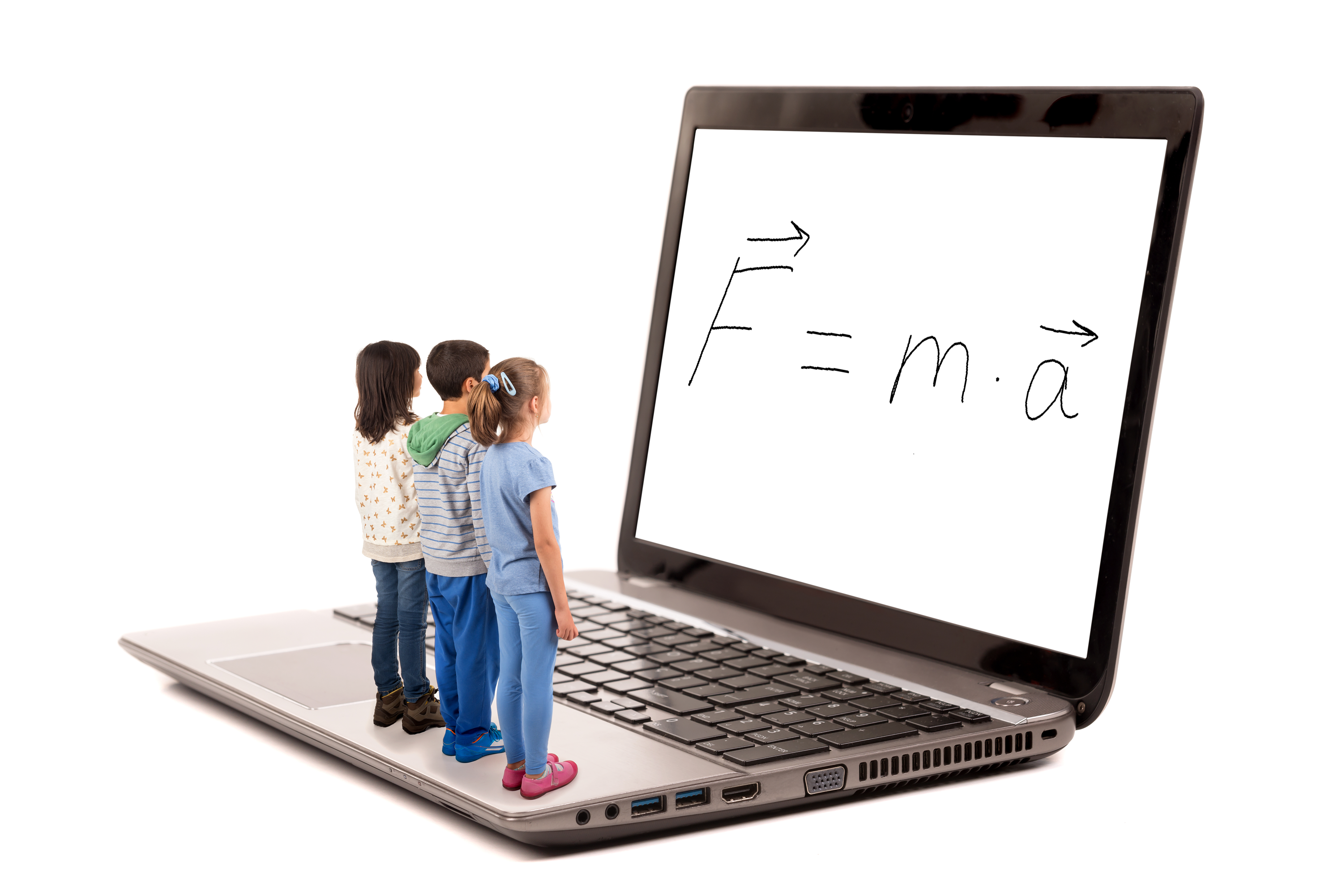The Independent Center for Integrative Education: Learning without Limits

Do You Speak Math?

What: An online course for students, interested in understanding what math is really about.Course Description

Traditionally, math is taught as a set of rules, symbols, theorems, exercises and awkward "word problems" pretending to be about real life. We would like to introduce it as what it really is: a compact and powerful language to describe simplified models of reality. Historically, those models came mainly from physics and moved on to engineering, chemistry, games, economics, computer science etc. This "lingistic" approach makes it easier to appreciate math and science by people with a "humanitarian brain."

Showing how the most basic physical models begot specific linguistic tools makes use of those tools intuitive, replacing memorization with common-sense thinking and understanding. Knowing the motivation for a tool, students can even re-invent it! With this approach, there is no need to oversimplify concepts or sacrifice rigor of statements and methods. Our experience shows that even the most abstract, rigorous, advanced and powerful mathematical concepts introduced in the right context cause no confusion and are easily understood by the students. Without overloading the student’s cognitive abilities, we will naturally introduce, use, and solve problems in set theory, logic, number theory, calculus, analytical geometry, linear algebra, trigonometry, theory of functions, differential equations, and much more.

Physics in this class is also introduced differently from its traditional image. We focus on the method of physics, which is creating and analyzing simplified models of natural phenomena. The simplification means abstracting from everything that can be neglected without throwing away the features of interest, which depend not only on the phenomenon studied, but also on the types of questions people ask about it. For that purpose, people created a mathematical language to describe those models and draw predictions that can be experimentally verified. Therefore, in this class we approach some areas that are not typically associated with physics, such as some topics in geometry, along the same lines as other physical models.

Homework in this course offers two types of tasks: exercises, that are necessary to build up fluency when studying any language, math included; and problems, that are integral part of the course because many important mathematical concepts and methods are introduced there. Skipping the homework will not prevent students from participation in further classes. However, if they skip the exercises, their ability to follow will be reduced due to lack of fluency in the language we will use, and skipping the problems will deprive the students from learning about many important physical concepts and mathematical techniques. The answers, solutions, and explanations are provided for all exercises and problems.

The target audience for this course is children ages about 12 and up. They will acquire skills and imagery from which they will draw analogies and concepts. That will help them solve problems in any area they will become interested in the future, be it science, technology, or humanities.

Syllabus

Classes are offered online for groups of 5 to 9 students. Those who are ready to commute to Bergen County, NJ, are welcome to participate in person. The duration of each class is 90 min, which includes a 10-min break in the middle. The syllabus is subject to change to adjust to specific questions and interests expressed by the students during the course.

Over the ten weekly classes of first part of the course, we will discuss the following most basic physical models:

Topic I. Discrete object model. (3 classes)

1. Countable and uncountable nouns in natural languages
2. Discrete or continuous? Not clear without a context.
3. Identifying (naming) objects.
4. Classes
5. Sets
6. Enumeration (natural numbers)
7. Counting objects (whole numbers)
8. Counting steps (integer numbers)
9. Dividing objects (rational numbers)
10. Symbolic expressions. Three meanings of the equal sign.
11. Isomorphism: a most powerful tool in problem solving
12. Measurements, units and errors. Dimensionality analysis.
13. Limits as a guarantee that measurement is possible with any accuracy

Topic II. Model of a point (2 classes)

1. Context: an object which is far away.
2. The only attribute: position
3. Ambiguity of terms. Point as an object, point as a position, point as a central idea, etc. Role of context.
4. Coordinates.
5. Points on a line. Irrational numbers. Real numbers.
6. Numbers and points: algebra-geometry dualism
7. Dimensions and degrees of freedom
8. The only possible operation: displacement (vectors).
10. Displacement and distance
11. Vector components
12. Operations on vectors: rotation and scaling
13. Vector operations in 2D: complex numbers
14. Vector operations in nD: introduction to linear algebra.

Topic III. Motion: adding time to position (2 classes)

1. Concept of time and its evolution
2. Model of time
3. Measuring time
4. Trajectory (position of a point as a function of time)
5. Frames of reference
6. Rates of change (derivatives)
7. Velocity and acceleration (generalization of vectors)
8. Reverse problem (integrals)
9. Average velocity
10. Velocity and speed

Topic IV. Point mass (3 classes)

1. What makes objects move? Four inseparable laws of Newton
2. First law: Inertia and inertial frames of reference
3. Second law: masses and forces
4. Third law: why mass is all we need to know to predict motion.
5. Law of gravity: why inertial frames of reference exist
6. First law as ordinary differential equation (ODE)
7. Free fall in the vicinity of Earth surface
8. Free fall far away from Earth surface (orbital motion). Astronomy.
9. Small parameter, Taylor series, first non-vanishing term
10. Hook's law, Ideal pendulum, Oscillations (trigonometry)
11. Liquid friction (exponential decay)
12. Unstable equilibrium (exponential growth)